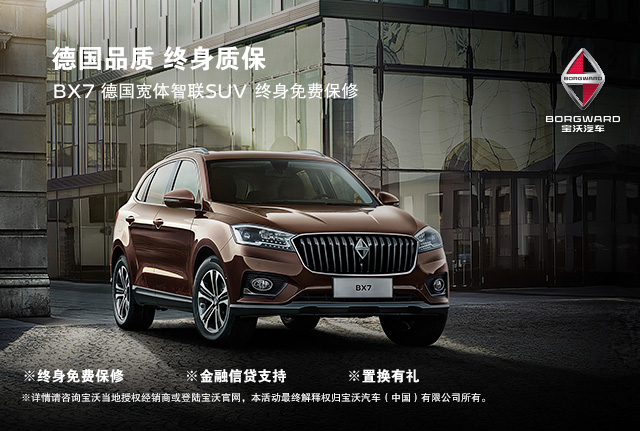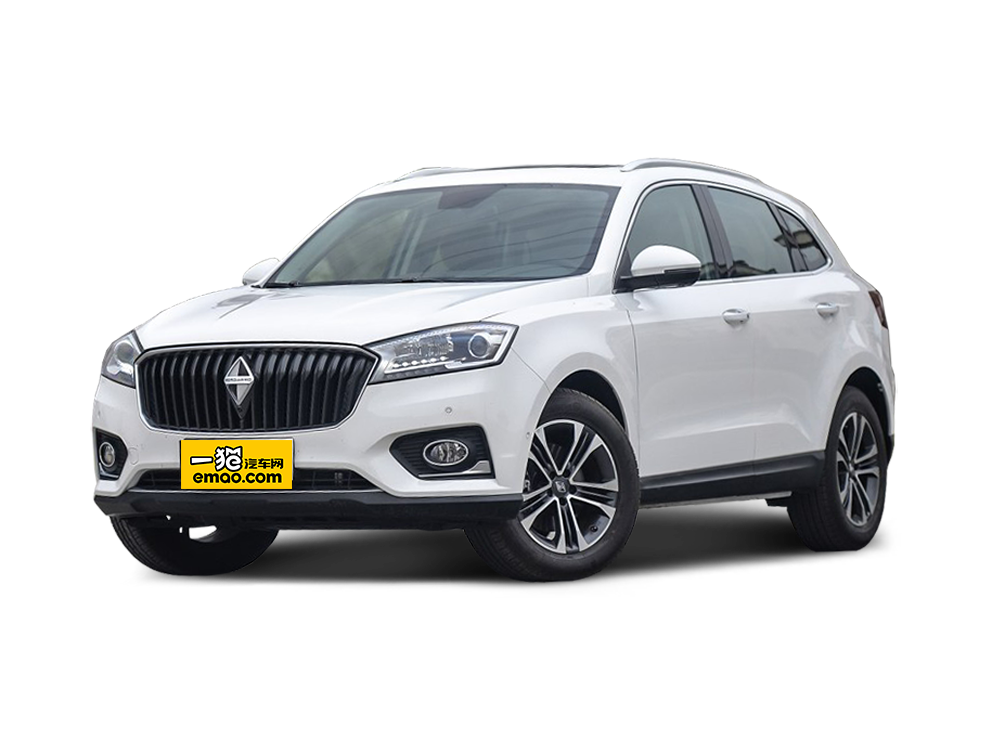当下，国内汽车市场已步入微增长时代，在政策、环境等因素影响下，豪华车市场增速不断放缓，竞争激烈程度与日俱增。日前，宝沃通过一项 “终身免费保修政策”对豪华做出了自己的诠释。

根据本项政策规定，首任宝沃BX7车主享受终身免费保修服务的同时，在整车保修期内还可享受3年不限里程免费道路救援服务的尊享服务。此外，宝沃汽车还推出了分期购买宝沃BX7即可尊享12/18期零利率政策，以及置换有礼政策，即任意品牌或者车型置换宝沃BX7均可享有高额现金补贴等市场促销政策。

在业内几乎没有先例的“终身免费保修”体现了宝沃对消费者的诚意，但同时也让这个国内车市的后来者面临不小的成本风险。这一服务政策的提出，则是宝沃汽车打破常规，让所有BX7车主均可享受到“终身免费保修”这一极具诚意的服务政策。同时也充分彰显出宝沃汽车对其BX7品质的高度自信。

针对这一政策的实施，宝沃(中国)副总裁兼营销公司总经理贾亚权表示：“为消费者打造高品质的德系汽车，并为其提供极具诚意的尊享服务，解决消费者后顾之忧是德国宝沃汽车的一贯宗旨。我们希望通过这一服务政策，展现我们对宝沃汽车的品质自信，同时也希望通过这一举措，让消费者实现终身用车无虑”。

作为宝沃汽车回归市场后的首款产品，宝沃BX7肩负着打响宝沃品牌复兴第一枪的重任。宝沃BX7定位为“德国宽体智联SUV”，车身尺寸达到4715mm、1910mm、1690mm，用数据很好地诠释了“宽体”的设计;在智能配置方面，宝沃BX7也是一部互联网汽车。通过B-Cloud宝沃云，以大数据为基础，同时转动“消费链+”和“产业链+”，实现从传统B2B2C到以用户为中心的B2C2B智能用车生态服务。最终可使用户具备“人”、“车” 两张超级身份证，可贯穿人和车的全生命周期管理。通过“九屏一云”服务平台，即TV终端、PC终端、手机终端、车机终端、销售终端、售后服务、生产终端、呼叫终端、总监控终端的智能联动，实践数字化B2C，带领用户快速进入更智能、更互联、更生态的大数据车生活时代。

据统计，今年7月正式启动销售以来，仅仅4个多月的时间里，宝沃BX7已累计收获了3.5万台订单，其中20万元以上高配车型的比例高达85%，而两驱与四驱的比例也接近1∶1,市场的卓越表现，进一步验证了市场对宝沃汽车产品及服务层面的高度认可。

为打造高品质汽车，宝沃自产品研发伊始便遵循严苛的德国的生产工艺、德国制造体系以及德国质量管理标准。为了保证产品品质，在中国建立了拥有全球首条八车型柔性生产线的 智能工厂，工厂融合了德国“汽车工业4.0”理念，采用了德国SAP、KUKA等领先的数字技术及智能化生产技术，以全新的智能制造模式打造出性能卓越、品质可靠的汽车。

2016年，宝沃汽车从新产品的发布到渠道布局建设，再到智能工厂的建设，从产品、品牌到本地化生产，宝沃用实力展现出企业的自信。而此次推出“终身免费保修政策”则进一步展现出宝沃汽车的诚意。未来，通过宝沃汽车环环相扣的体系建设，在全球布局中宝沃必将锐意前行，以品质、诚意获得全球消费者的信赖。

|分享

•已阅！握爪
•我手滑为你点赞
•128个赞！
•不明觉厉
•阅后既醉
•有钱！任性
•照片太美，我不敢看
•干货！杠杠的
•高大上
•膜拜中！
•请接受我的膝盖
•猫哥/猫妹么么哒
•神吐槽
•我只笑笑不说话
•我想静静
•窒息
##### 资讯相关车系##### 热度排行• 阿斯顿·马丁
• 奥迪
• 阿尔法罗密欧
• ALPINA
• 爱驰
• AC Schnitzer

• 巴博斯
• 宝骏
• 宝马
• 保时捷
• 北汽制造
• 奔驰
• 奔腾
• 本田
• 比亚迪
• 标致
• 别克
• 宾利
• 布加迪
• 北汽威旺
• 北京
• 北汽绅宝
• 北汽幻速
• 北汽新能源
• 宝沃
• 比速汽车
• 北汽道达
• 北京汽车
• 比德文汽车
• 铂驰
• 北汽昌河

• 昌河
• 长安
• 长城
• 长安商用
• 成功汽车
• 长江EV
• 长安轻车型
• 长安欧尚
• 长安凯程
• 长安跨越
• 车驰汽车

• 大众
• 道奇
• 东风
• 东风风神
• 东风小康
• 东南
• DS
• 东风风行
• 东风风度
• 东风风光
• 电咖
• 大乘汽车
• 大运
• 东风富康

• 法拉利
• 菲亚特
• 丰田
• 福迪
• 福特
• 福田汽车
• 福汽启腾
• 风诺
• 福田

• GMC
• 广汽传祺
• 广汽吉奥
• 观致
• 国金汽车
• 广汽新能源
• 广汽集团
• 国机智骏

• 哈飞
• 海格
• 海马
• 华泰
• 黄海
• 恒天
• 红旗
• 哈弗
• 华颂
• 华凯
• 华泰新能源
• 汉腾汽车
• 华利
• 红星汽车
• HYCAN合创
• 汉龙汽车

• Jeep
• 江淮
• 江铃
• 捷豹
• 金杯
• 九龙
• 吉利汽车
• 金旅
• 金龙
• 江铃集团轻汽
• 江铃集团新能源
• 君马汽车
• 捷途
• 钧天
• 捷达
• 几何汽车

• 开瑞
• 凯迪拉克
• 科尼赛克
• 克莱斯勒
• KTM
• 卡威
• 凯翼
• 康迪
• 康迪电动汽车集团
• 开沃汽车
• 卡升

• 猎豹汽车
• 兰博基尼
• 劳斯莱斯
• 雷克萨斯
• 雷诺
• 理念
• 力帆
• 莲花汽车
• 林肯
• 铃木
• 陆风
• 路虎
• 路特斯
• 领志
• 领克
• 零跑汽车
• Lorinser
• LOCAL MOTORS
• LITE
• 领途汽车
• 理想汽车
• 罗夫哈特
• 力帆汽车

• MG
• MINI
• 马自达
• 玛莎拉蒂
• 迈凯伦
• 摩根
• 名爵
• 迈莎锐
• 迈迈

• 纳智捷
• 哪吒汽车

• 讴歌
• 欧宝
• 欧朗
• 欧拉
• 欧尚汽车

• 帕加尼
• Polestar极星

• 奇瑞
• 启辰
• 起亚
• 前途
• 庆铃汽车
• 骐铃汽车
• 全球鹰
• 乔治·巴顿

• 日产
• 荣威
• 瑞麒汽车
• 如虎
• 瑞驰
• 瑞麒
• 瑞驰新能源
• 容大智造

• 上汽大通
• smart
• 三菱
• 双环
• 双龙
• 斯巴鲁
• 斯柯达
• 萨博
• 思铭
• 赛麟
• SWM斯威汽车
• 上汽MAXUS
• SRM鑫源
• 思皓
• SHELBY
• 上喆

• TESLA
• 泰卡特
• 腾势
• 特斯拉
• 天际汽车

• 威麟
• 威兹曼
• 沃尔沃
• 五菱汽车
• 五十铃
• 潍柴英致
• WEY
• 蔚来
• 威马汽车
• 潍柴汽车

• 现代
• 雪佛兰
• 雪铁龙
• 西雅特
• 新特汽车
• 小鹏汽车
• 新宝骏

• 野马汽车
• 一汽
• 依维柯
• 英菲尼迪
• 永源
• 驭胜
• 云度
• 宇通客车
• 云雀汽车
• 远程汽车
• 一汽凌河

• 中华
• 中兴
• 众泰
• 知豆
• 之诺
• 正道汽车
• A
• B
• C
• D
• E
• F
• G
• H
• I
• J
• K
• L
• M
• N
• O
• P
• Q
• R
• S
• T
• U
• V
• W
• X
• Y
• Z

• 阿斯顿·马丁
• 奥迪
• 阿尔法罗密欧
• ALPINA
• 爱驰
• AC Schnitzer

• 巴博斯
• 宝骏
• 宝马
• 保时捷
• 北汽制造
• 奔驰
• 奔腾
• 本田
• 比亚迪
• 标致
• 别克
• 宾利
• 布加迪
• 北汽威旺
• 北京
• 北汽绅宝
• 北汽幻速
• 北汽新能源
• 宝沃
• 比速汽车
• 北汽道达
• 北京汽车
• 比德文汽车
• 铂驰
• 北汽昌河

• 昌河
• 长安
• 长城
• 长安商用
• 成功汽车
• 长江EV
• 长安轻车型
• 长安欧尚
• 长安凯程
• 长安跨越
• 车驰汽车

• 大众
• 道奇
• 东风
• 东风风神
• 东风小康
• 东南
• DS
• 东风风行
• 东风风度
• 东风风光
• 电咖
• 大乘汽车
• 大运
• 东风富康

• 法拉利
• 菲亚特
• 丰田
• 福迪
• 福特
• 福田汽车
• 福汽启腾
• 风诺
• 福田

• GMC
• 广汽传祺
• 广汽吉奥
• 观致
• 国金汽车
• 广汽新能源
• 广汽集团
• 国机智骏

• 哈飞
• 海格
• 海马
• 华泰
• 黄海
• 恒天
• 红旗
• 哈弗
• 华颂
• 华凯
• 华泰新能源
• 汉腾汽车
• 华利
• 红星汽车
• HYCAN合创
• 汉龙汽车

• Jeep
• 江淮
• 江铃
• 捷豹
• 金杯
• 九龙
• 吉利汽车
• 金旅
• 金龙
• 江铃集团轻汽
• 江铃集团新能源
• 君马汽车
• 捷途
• 钧天
• 捷达
• 几何汽车

• 开瑞
• 凯迪拉克
• 科尼赛克
• 克莱斯勒
• KTM
• 卡威
• 凯翼
• 康迪
• 康迪电动汽车集团
• 开沃汽车
• 卡升

• 猎豹汽车
• 兰博基尼
• 劳斯莱斯
• 雷克萨斯
• 雷诺
• 理念
• 力帆
• 莲花汽车
• 林肯
• 铃木
• 陆风
• 路虎
• 路特斯
• 领志
• 领克
• 零跑汽车
• Lorinser
• LOCAL MOTORS
• LITE
• 领途汽车
• 理想汽车
• 罗夫哈特
• 力帆汽车

• MG
• MINI
• 马自达
• 玛莎拉蒂
• 迈凯伦
• 摩根
• 名爵
• 迈莎锐
• 迈迈

• 纳智捷
• 哪吒汽车

• 讴歌
• 欧宝
• 欧朗
• 欧拉
• 欧尚汽车

• 帕加尼
• Polestar极星

• 奇瑞
• 启辰
• 起亚
• 前途
• 庆铃汽车
• 骐铃汽车
• 全球鹰
• 乔治·巴顿

• 日产
• 荣威
• 瑞麒汽车
• 如虎
• 瑞驰
• 瑞麒
• 瑞驰新能源
• 容大智造

• 上汽大通
• smart
• 三菱
• 双环
• 双龙
• 斯巴鲁
• 斯柯达
• 萨博
• 思铭
• 赛麟
• SWM斯威汽车
• 上汽MAXUS
• SRM鑫源
• 思皓
• SHELBY
• 上喆

• TESLA
• 泰卡特
• 腾势
• 特斯拉
• 天际汽车

• 威麟
• 威兹曼
• 沃尔沃
• 五菱汽车
• 五十铃
• 潍柴英致
• WEY
• 蔚来
• 威马汽车
• 潍柴汽车

• 现代
• 雪佛兰
• 雪铁龙
• 西雅特
• 新特汽车
• 小鹏汽车
• 新宝骏

• 野马汽车
• 一汽
• 依维柯
• 英菲尼迪
• 永源
• 驭胜
• 云度
• 宇通客车
• 云雀汽车
• 远程汽车
• 一汽凌河

• 中华
• 中兴
• 众泰
• 知豆
• 之诺
• 正道汽车
• A
• B
• C
• D
• E
• F
• G
• H
• I
• J
• K
• L
• M
• N
• O
• P
• Q
• R
• S
• T
• U
• V
• W
• X
• Y
• Z

• 阿斯顿·马丁
• 奥迪
• 阿尔法罗密欧
• ALPINA
• 爱驰
• AC Schnitzer

• 巴博斯
• 宝骏
• 宝马
• 保时捷
• 北汽制造
• 奔驰
• 奔腾
• 本田
• 比亚迪
• 标致
• 别克
• 宾利
• 布加迪
• 北汽威旺
• 北京
• 北汽绅宝
• 北汽幻速
• 北汽新能源
• 宝沃
• 比速汽车
• 北汽道达
• 北京汽车
• 比德文汽车
• 铂驰
• 北汽昌河

• 昌河
• 长安
• 长城
• 长安商用
• 成功汽车
• 长江EV
• 长安轻车型
• 长安欧尚
• 长安凯程
• 长安跨越
• 车驰汽车

• 大众
• 道奇
• 东风
• 东风风神
• 东风小康
• 东南
• DS
• 东风风行
• 东风风度
• 东风风光
• 电咖
• 大乘汽车
• 大运
• 东风富康

• 法拉利
• 菲亚特
• 丰田
• 福迪
• 福特
• 福田汽车
• 福汽启腾
• 风诺
• 福田

• GMC
• 广汽传祺
• 广汽吉奥
• 观致
• 国金汽车
• 广汽新能源
• 广汽集团
• 国机智骏

• 哈飞
• 海格
• 海马
• 华泰
• 黄海
• 恒天
• 红旗
• 哈弗
• 华颂
• 华凯
• 华泰新能源
• 汉腾汽车
• 华利
• 红星汽车
• HYCAN合创
• 汉龙汽车

• Jeep
• 江淮
• 江铃
• 捷豹
• 金杯
• 九龙
• 吉利汽车
• 金旅
• 金龙
• 江铃集团轻汽
• 江铃集团新能源
• 君马汽车
• 捷途
• 钧天
• 捷达
• 几何汽车

• 开瑞
• 凯迪拉克
• 科尼赛克
• 克莱斯勒
• KTM
• 卡威
• 凯翼
• 康迪
• 康迪电动汽车集团
• 开沃汽车
• 卡升

• 猎豹汽车
• 兰博基尼
• 劳斯莱斯
• 雷克萨斯
• 雷诺
• 理念
• 力帆
• 莲花汽车
• 林肯
• 铃木
• 陆风
• 路虎
• 路特斯
• 领志
• 领克
• 零跑汽车
• Lorinser
• LOCAL MOTORS
• LITE
• 领途汽车
• 理想汽车
• 罗夫哈特
• 力帆汽车

• MG
• MINI
• 马自达
• 玛莎拉蒂
• 迈凯伦
• 摩根
• 名爵
• 迈莎锐
• 迈迈

• 纳智捷
• 哪吒汽车

• 讴歌
• 欧宝
• 欧朗
• 欧拉
• 欧尚汽车

• 帕加尼
• Polestar极星

• 奇瑞
• 启辰
• 起亚
• 前途
• 庆铃汽车
• 骐铃汽车
• 全球鹰
• 乔治·巴顿

• 日产
• 荣威
• 瑞麒汽车
• 如虎
• 瑞驰
• 瑞麒
• 瑞驰新能源
• 容大智造

• 上汽大通
• smart
• 三菱
• 双环
• 双龙
• 斯巴鲁
• 斯柯达
• 萨博
• 思铭
• 赛麟
• SWM斯威汽车
• 上汽MAXUS
• SRM鑫源
• 思皓
• SHELBY
• 上喆

• TESLA
• 泰卡特
• 腾势
• 特斯拉
• 天际汽车

• 威麟
• 威兹曼
• 沃尔沃
• 五菱汽车
• 五十铃
• 潍柴英致
• WEY
• 蔚来
• 威马汽车
• 潍柴汽车

• 现代
• 雪佛兰
• 雪铁龙
• 西雅特
• 新特汽车
• 小鹏汽车
• 新宝骏

• 野马汽车
• 一汽
• 依维柯
• 英菲尼迪
• 永源
• 驭胜
• 云度
• 宇通客车
• 云雀汽车
• 远程汽车
• 一汽凌河

• 中华
• 中兴
• 众泰
• 知豆
• 之诺
• 正道汽车
• A
• B
• C
• D
• E
• F
• G
• H
• I
• J
• K
• L
• M
• N
• O
• P
• Q
• R
• S
• T
• U
• V
• W
• X
• Y
• Z